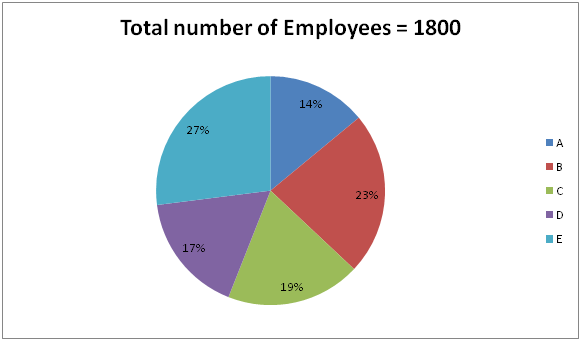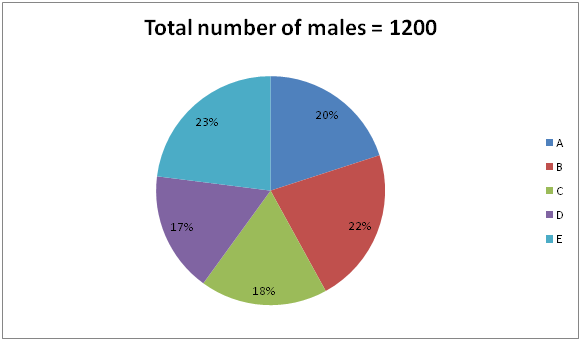# SBI PO Prelims Quantitative Aptitude Questions 2019 (Day-43)

Dear Aspirants, Our IBPS Guide team is providing new series of Quantitative Aptitude Questions for SBI PO 2019 so the aspirants can practice it on a daily basis. These questions are framed by our skilled experts after understanding your needs thoroughly. Aspirants can practice these new series questions daily to familiarize with the exact exam pattern and make your preparation effective.

[WpProQuiz 6328]

### Click Here for SBI PO Pre 2019 High-Quality Mocks Exactly on SBI Standard

Directions (Q. 1 – 5): What value should come in place of (?) in the following questions?

1) (11/110) of 54330 + 6.5 ÷ 13 × 280 – 31 × 5 = ?

a) 5418

b) 5952

c) 4276

d) 4534

e) None of these

2) 1680 ÷ ? + 173 × 2 = 642 – 256 + 25 % of 400

a) 18

b) 23

c) 15

d) 12

e) None of these

3) (242 – 112) × 8 ÷ 26 + ? % of 250 = 196

a) 28

b) 22.4

c) 19.6

d) 33

e) None of these

4) ? % of (√144) × 25 + 390 = 453

a) 28

b) 32

c) 21

d) 17

e) None of these

5) (8/11) of [956 – 373] + 286 = ? – 1125

a) 1835

b) 2156

c) 2278

d) 1543

e) None of these

Directions (6 – 10): Study the following information carefully and answer the questions given below.

Following two pie chart show the information about total number of employees and total number of male employees in 5 different companies respectively.6) If the ratio of females who are under graduate and post graduate in company B and C is 7: 8 and 13: 8 respectively, total number of females who are post graduate in those company is approximately what percentage of number of males in those company?

a) 37%

b) 23%

c) 25%

d) 29%

e) 27%

7) Find the ratio between the average number of female in company A, D and E together to the number of employees in E.

a) 1: 2

b) 2: 3

c) 3: 7

d) 2: 9

e) 1: 9

8) Find the impact on total number of employees in company C and D, if the number of males in company C and D increased by 50%.

a) 28% increase

b) 32% increase

c) 35% increase

d) 56% increase

e) 15% increase

9) Find the ratio between the sum of the 15% of male employees in company A and 18% of female employees in company B and the sum of total employees in C and the number of male employees in D.

a) 26: 5

b) 2: 15

c) 3: 13

d) 3: 26

e) 15: 7

10) What is the difference between the central angle made by the employees of A, C and E and the number of females in company C, D and E, if the total number of females are put in a pie chart?

a) 46.8°

b) 36.5°

c) 56.2°

d) 24.9°

e) 85.6°

(11/110)*54330 + (6.5/13)* 280 – 31*5 = x

5433 + 140 – 155 = x

X = 5418

(1680/x) + 173*2 = 642 – 256 + (25/100)*400

(1680/x) = 642 – 256 + 100 – 346

(1680/x) = 140

X = 12

[(24 + 11) (24 – 11)] × 8 ÷ 26 + (x/100)*250 = 196

(35*13*8)/26 + (5x/2) = 196

140 + (5x/2) = 196

(5x/2) = 196 – 140

5x/2 = 56

X = (56*2)/5 = 22.4

(X/100)*12*25 + 390 = 453

3x = 453 – 390

3x = 63

X = 63/3 = 21

(8/11)*583 + 286 = x – 1125

424 + 286 + 1125 = x

X = 1835

Directions (6 – 10):

In B,

Total number of employees = 23/100 * 1800 = 414

Number of males = 22/100 * 1200 = 264

Number of females = 414 – 264 = 150

Number of females who are post graduate = 150/15 * 8 = 80

In C,

Total number of employees = 19/100 * 1800 = 342

Number of males = 18/100 * 1200 = 216

Number of females = 342 – 216 = 126

Number of females who are post graduate = 126/21 * 8 = 48

Required % = [(80 + 48)/(264 + 216)] * 100 = 128/480 * 100 = 26.667% = 27%

In A,

Total number of employees = 14/100 * 1800 = 252

Number of males = 20/100 * 1200 = 240

Number of females = 252 – 240 = 12

In D,

Total number of employees = 17/100 * 1800 = 306

Number of males = 17/100 * 1200 = 204

Number of females = 306 – 204 = 102

In E,

Total number of employees = 27/100 * 1800 = 486

Number of males = 23/100 * 1200 = 276

Number of females = 486 – 276 = 210

Required ratio = [(12 + 102 + 210)/3]: 486

= 2: 9

In D,

Total number of employees = 17/100 * 1800 = 306

Number of males = 17/100 * 1200 = 204

50% of males = 102

In C,

Total number of employees = 19/100 * 1800 = 342

Number of males = 18/100 * 1200 = 216

50% of males = 108

Total increase = 210

% of increase = (210/(342 + 306)) * 100 = 32.40%  = 32%

In A,

Number of males = 20/100 * 1200 = 240

15% of males =240 * 15/100 = 36

In B,

Total number of employees = 23/100 * 1800 = 414

Number of males = 22/100 * 1200 = 264

Number of females = 414 – 264 = 150

18% of females = 150 * 18/100 = 27

In D,

Number of males = 17/100 * 1200 = 204

In C,

Total number of employees = 19/100 * 1800 = 342

Required ratio = (36 + 27): (342 + 204) = 3: 26

In A,

Total number of employees = 14/100 * 1800 = 252

In C,

Total number of employees = 19/100 * 1800 = 342

Number of males = 18/100 * 1200 = 216

Number of females = 342 – 216 = 126

In D,

Total number of employees = 17/100 * 1800 = 306

Number of males = 17/100 * 1200 = 204

Number of females = 306 – 204 = 102

In E,

Total number of employees = 27/100 * 1800 = 486

Number of males = 23/100 * 1200 = 276

Number of females = 486 – 276 = 210

Total female employees = 1800 – 1200 = 600 = 360°

Total female employees in C, D and E = 126 + 102 + 210 = 438

Angle made by female employees in C, D and E = (360 * 438)/600

= 262.8°

Angle made by employees in A, C and E = [(14 + 19 + 27)/100] * 360

= 216°

Required difference = 262.8° – 216°

= 46.8°

Related Articles :

SBI PO Prelims Quantitative Aptitude Questions 2019 (Day-42)